Capacitor and Capacitance
2022-12-07

A capacitor is a little like a battery but works completely differently. A battery is an electronic device that converts chemical energy into electrical energy, whereas a capacitor is an electronic component that stores electrostatic energy in an electric field.

What is a capacitor?

A capacitor is a two-terminal electrical device that can store energy in the form of an electric charge. It consists of two electrical conductors that are separated by a distance.  The space between the conductors may be filled by vacuum or with an insulating material known as a dielectric. The ability of the capacitor to store charges is known as capacitance.

Capacitors store energy by holding apart pairs of opposite charges. The simplest design for a capacitor is a parallel plate, which consists of two metal plates with a gap between them. But, different types of capacitors are manufactured in many forms, styles, lengths, girths, and materials.

How does a capacitor work?

For demonstration, let us consider the most basic structure of a capacitor – the parallel plate capacitor. It consists of two parallel plates separated by a dielectric. When we connect a DC voltage source across the capacitor, one plate is connected to the positive end (plate I) and the other to the negative end (plate II). When the potential of the battery is applied across the capacitor, plate I become positive with respect to plate II. The current tries to flow through the capacitor at the steady-state condition from its positive plate to its negative plate. But it cannot flow due to the separation of the plates with an insulating material.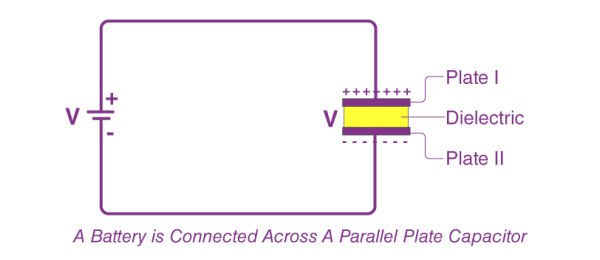An electric field appears across the capacitor. The positive plate (plate I) accumulates positive charges from the battery, and the negative plate (plate II) accumulates negative charges from the battery.  After a point, the capacitor holds the maximum amount of charge as per its capacitance with respect to this voltage. This time span is called the charging time of the capacitor.

When the battery is removed from the capacitor, the two plates hold a negative and positive charge for a certain time. Thus, the capacitor acts as a source of electrical energy.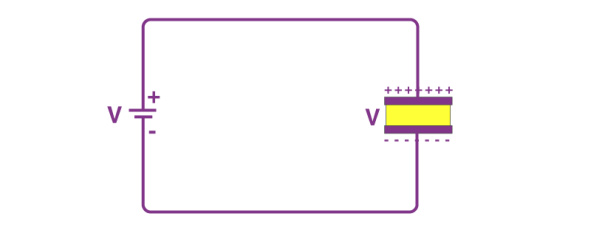If these plates are connected to a load, the current flows to the load from Plate I to Plate II until all the charges are dissipated from both plates. This time span is known as the discharging time of the capacitor.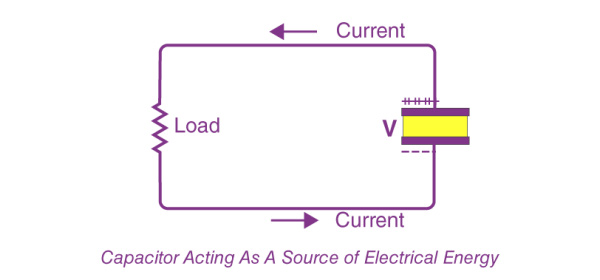How do you determine the value of capacitance?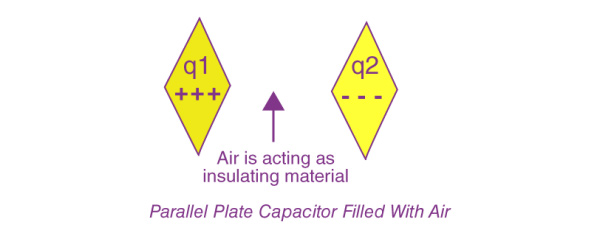The conducting plates have some charges Q1 and Q2 (Usually, if one plate has +q, the other has –q charge). The electric field in the region between the plates depends on the charge given to the conducting plates. We also know that potential difference (V) is directly proportional to the electric field hence we can say,

Q∝V

Q=CV

C=Q/V

This constant of proportionality is known as the capacitance of the capacitor.

Capacitance is the ratio of the change in the electric charge of a system to the corresponding change in its electric potential.

The capacitance of any capacitor can be either fixed or variable, depending on its usage. From the equation, it may seem that ‘C’ depends on charge and voltage. Actually, it depends on the shape and size of the capacitor and also on the insulator used between the conducting plates.

Energy stored in a capacitor

Once the opposite charges have been placed on either side of a parallel-plate capacitor, the charges can be used to work by allowing them to move towards each other through a circuit. The equation gives the total energy that can be extracted from a fully charged capacitor:

U=½CV*2

Capacitors function a lot like rechargeable batteries. The main difference between a capacitor and a battery lies in the technique they employ to store energy. Unlike batteries, the capacitor’s ability to store energy doesn’t come from chemical reactions but from the physical design that allows it to hold negative and positive charges apart.

Standard units of capacitance

Most of the electrical and electronic applications are covered by the following standard unit (SI) prefixes for easy calculations:

· 1 mF (millifarad) = 10−3 F

· 1 μF (microfarad) =10−6 F

· 1 nF (nanofarad) = 10−9 F

· 1 pF (picofarad) = 10−12 F

Capacitance of a parallel plate capacitor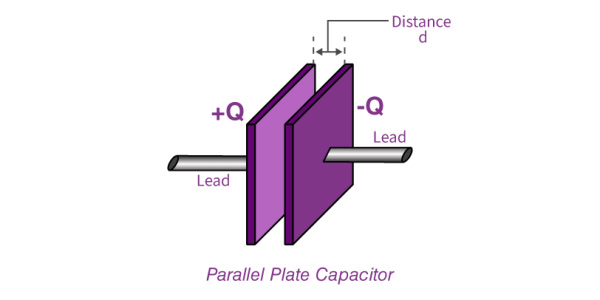The parallel plate capacitor as shown in the figure has two identical conducting plates, each having a surface area A and separated by a distance d. When voltage V is applied to the plates, it stores charge Q.

The force between charges increases with charge values and decreases with the distance between them. The bigger the area of the plates, the more charge they can store.  Hence, the value of C is greater for a large value of A. Similarly, the closer the plates are, the greater the attraction of the opposite charges on them. Therefore C is greater for a smaller d.

The formula gives the charge density on the plates

σ=Q/A

When the distance of separation (d) is small, the electric field between the plates is fairly uniform, and its magnitude is given by:

E=σ/ϵ0

As the electric field between the plates is uniform, the potential difference between the plates is given by

V=Ed=σd/ϵ0=Qd/ϵ0A

Substituting the above value of V in the capacitance formula, we get

C=Q/V=Q/(Qd/ϵ0A)=ϵ0 A/d

 The capacitance of a parallel plate capacitor is given by the formula C=ϵ0 A/d

Capacitance of a spherical capacitor

Spherical capacitors consist of two concentric conducting spherical shells of radii  R1 and R2. The shells are given equal and opposite charges +Q and –Q respectively. The electric field between shells is directed radially outward. The magnitude of the field can be obtained by applying Gauss law over a spherical Gaussian surface of radius r concentric with the shells.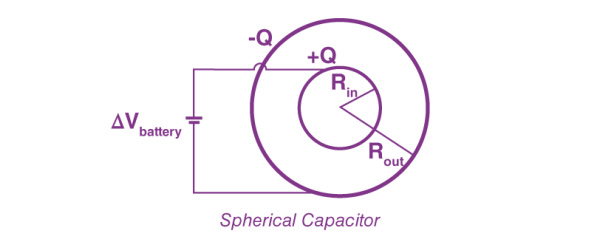The enclosed charge is +Q, therefore

∮SE→⋅n^dA=E(4πr2)=Q/ϵ0

The electric field between the conductor is given as

E→=(1/4πϵ0)*(Q/r2)*r^

Integrating E along the radial path between the shells, we get

V=∫R1R2E→⋅dl→=(Q/4πϵ0)(1/R1−1/R2)

The potential difference between two conductors can be calculated using the formula

VB−VA=−∫ABE→dL→

The potential difference between the plates is

V=−(V2−V1)=V1−V2

Substituting the value of V in the capacitance formula, we get

C=Q/V=4πϵ0*(R1R2/(R2−R1))

 The capacitance of a spherical capacitor is given by the equation C=4πϵ0*(R1R2/(R2−R1))

Factors affecting capacitance

Dielectric

The effect of dielectric on capacitance is that the greater the permittivity of the dielectric, the greater the capacitance, likewise lesser the permittivity of the dielectric the lesser is the capacitance. Some materials offer less opposition to the field flux for a given amount of field force. Materials with greater permittivity allow more field flux. Hence greater charge is collected.

Plate spacing

The effect of spacing on the capacitance is that it is inversely proportional to the distance between the plates. Mathematically it is given as:

C∝1d

Area of the plates

The effect of the area of the plate is that the capacitance is directly proportional to the area. The larger the plate area, the more the capacitance value. Mathematically it is given as:

C  A

What are the applications of capacitors?

Capacitors for energy storage

Since the late 18th century, capacitors have been used to store electrical energy. Individual capacitors do not hold much energy, providing only enough power for electronic devices during temporary power outages or when they need additional power. Many applications use capacitors as energy sources, and a few of them are as follows:

· Audio equipment

· Camera Flashes

· Power supplies

· Magnetic coils

· Lasers

Supercapacitors are capacitors that have high capacitances up to 2 kF. These capacitors store large amounts of energy and offer new technological possibilities in areas such as electric cars, regenerative braking in the automotive industry and industrial electrical motors, computer memory backup during power loss, and many others.

Capacitors for power conditioning

One of the important applications of capacitors is the conditioning of power supplies. Capacitors allow only AC signals to pass when they are charged, blocking DC signals. This capacitor effect is used in separating or decoupling different parts of electrical circuits to reduce noise as a result of improving efficiency. Capacitors are also used in utility substations to counteract inductive loading introduced by transmission lines.

Capacitors as sensors

Capacitors are used as sensors to measure a variety of things including humidity, mechanical strain, and fuel levels. Two aspects of capacitor construction are used in the sensing application – the distance between the parallel plates and the material between them. The former detects mechanical changes such as acceleration and pressure, and the latter is used in sensing air humidity.

Capacitors for signal processing

There are advanced applications of capacitors in information technology. Capacitors are used by Dynamic Random Access Memory (DRAM) devices to represent binary information as bits. Capacitors are also used in conjunction with inductors to tune circuits to particular frequencies, an effect exploited by radio receivers, speakers, and analog equalizers.

Frequently asked questions on capacitors and capacitance

What is a variable capacitor?

A variable capacitor is a capacitor whose capacitance can be varied to a certain range of values based on necessity. The two plates of the variable capacitor are made of metals where one of the plates is fixed, and the other is movable. Their main function is to fix the resonant frequency in the LC circuit. There are two types of variable frequency and they are, tunning capacitors and trimming capacitors.

How does the shape of the capacitor affect its capacitance?

The distance between the plates

The more distant the plates are, the less the free electrons on the far plate feel the push of the electrons that are being added to the negative plate. This makes it harder to add more negative charges to the negative plate. The current will flow through a short circuit if the plates are closer. This implies that the capacitance of a parallel plate is inversely related to the plate separation.

Area of the plates

It’s easier to add charges to a capacitor if the parallel plates have a huge area. Two wide metal plates would give two repelling-like charges a greater range to spread out across the plate, making it easier to add a lot more negative charges to one plate. Likewise, a very small plate area would cause the electrons to get cramped together earlier, making it harder to get a large difference in charge for a given voltage.

What are ultracapacitors?

An ultracapacitor, also known as the supercapacitor, is a high-capacity capacitor with a capacitance value much higher than other capacitors but with lower voltage limits.

How long does a capacitor last?

Capacitors have a limited life span. Most capacitors are designed to last approximately 20 years.

What kind of energy is stored in a capacitor?

Energy stored in a capacitor is electrical potential energy, thus related to the charge Q and voltage V on the capacitor.

Why isn’t water used as a dielectric in a capacitor?

Water has a high dielectric constant but a very low dielectric strength, hence it would act as a conductor and leak charges through it.

RECOMMENDED FOR YOU
GDHY Revolutionizing Capacitor Design to Enhance Performance and Efficiency in Small Welding and Quenching Equipment
Precautions for using film capacitors
Start capacitor in AC Induction Motor
How does metallized polyester film capacitors work in the application?
How to chose water cooled resonant capacitor ?
What is an axial film capacitor?
What is a plastic film capacitor?
IGBT Snubber Capacitors Selection Guide
What is a metallized polyester film capacitor?
What is a coupling capacitor and a bypass capacitor?
Get In Touch With Us
If you contact us now for more details, you can take samples for free. Our service team will get back to you with in 24 hours normally !